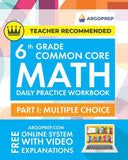•# 6th Grade Common Core Math: Daily Practice Workbook - Part I: Multiple Choice 1000+ Practice Questions and Video Explanations Argo Brothers (Common Core Math by ArgoPrep)

Argo Brothers Inc
SKU: 9781951048907
10 En stock

vista rápida

This book is your comprehensive workbook for 6th Grade Common Core Math. By practicing and mastering this entire workbook, your child will become very familiar and comfortable with the state math exam and common core standards. This 6th Grade Common Core Math Workbook (Multiple Choice) includes: 20 Weeks of Daily...
Precio regular \$15.78 Precio de venta Precio unitario: \$0.00

Pago seguro y confiable con

This book is your comprehensive workbook for 6th Grade Common Core Math. By practicing and mastering this entire workbook, your child will become very familiar and comfortable with the state math exam and common core standards. This 6th Grade Common Core Math Workbook (Multiple Choice) includes: 20 Weeks of Daily Multiple Choice Practice Weekly Assessments State Aligned Common Core Curriculum End of Year Assessment This book has the following topics covered: Week 1 - Dividing with fractions, solving word problems Week 2 - Dividing multi-digit, adding, subtracting, multiplying and dividing decimal numbers Week 3 - Greatest Common Factor and Least Common Multiple Week 4 - Coordinate Graphs Week 5 - Graph points that represent real numbers in real situations. Week 6 - Solving equations using the substitution method Week 7 - Rewriting numbers and algebraic expressions Week 8 - Understand solving an equation or inequality as a process of answering a question Week 9 - Practice writing your own algebraic expressions Week 10 - Write your own equations or inequalities to make them fit any situation you are given Week 11 - Understand how one variable may affect another variable Week 12 - Studying unit rates to solve real-world problems Week 13 - Percents & Statistics Week 14 - Analyzing data sets Week 15 - Understanding boxplots Week 16 - Interpreting graphs, charts, and tables Week 17 - Interpreting graphs, charts, and tables (continued) Week 18 - Practice finding the area of figures Week 19 - Calculate the volume of three-dimensional objects Week 20 - Coordinate plane & three-dimensional figures Each question is labeled with the specific common core standard so both parents and teachers can use this workbook for their student(s). This workbook takes the Common Core State Standards and divides them up among 20 weeks. By working on these problems on a daily basis, students will be able to (1) find any deficiencies in their understanding and/or practice of math and (2) have small successes each day that will build competence and confidence in their abilities.

• | Author: Argoprep
• | Publisher: Argo Brothers Inc
• | Publication Date: Nov 12, 2021
• | Number of Pages: 152 pages
• | Language: English
• | Binding: Paperback
• | ISBN-10: 1951048903
• | ISBN-13: 9781951048907

Su carrito está vacío